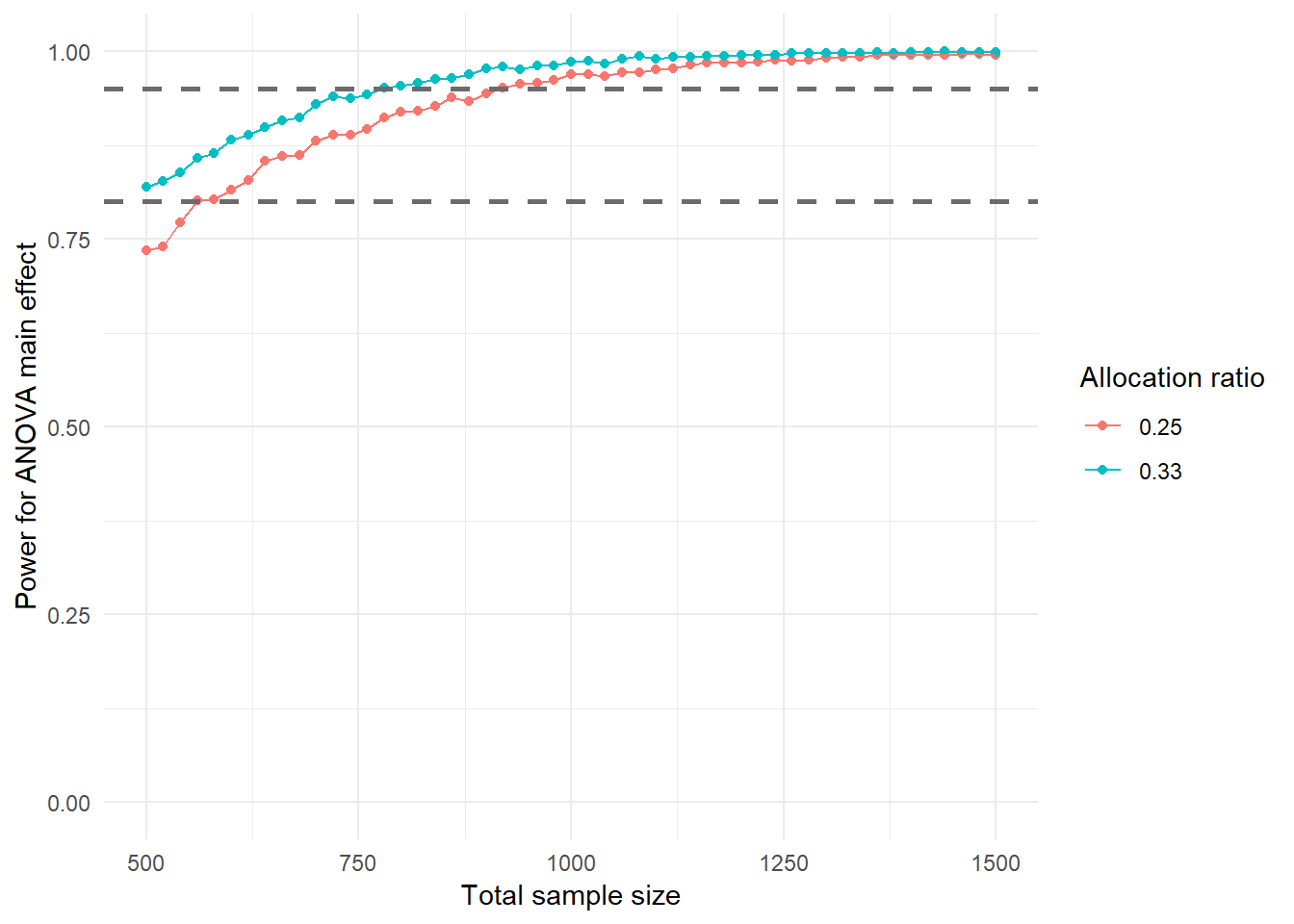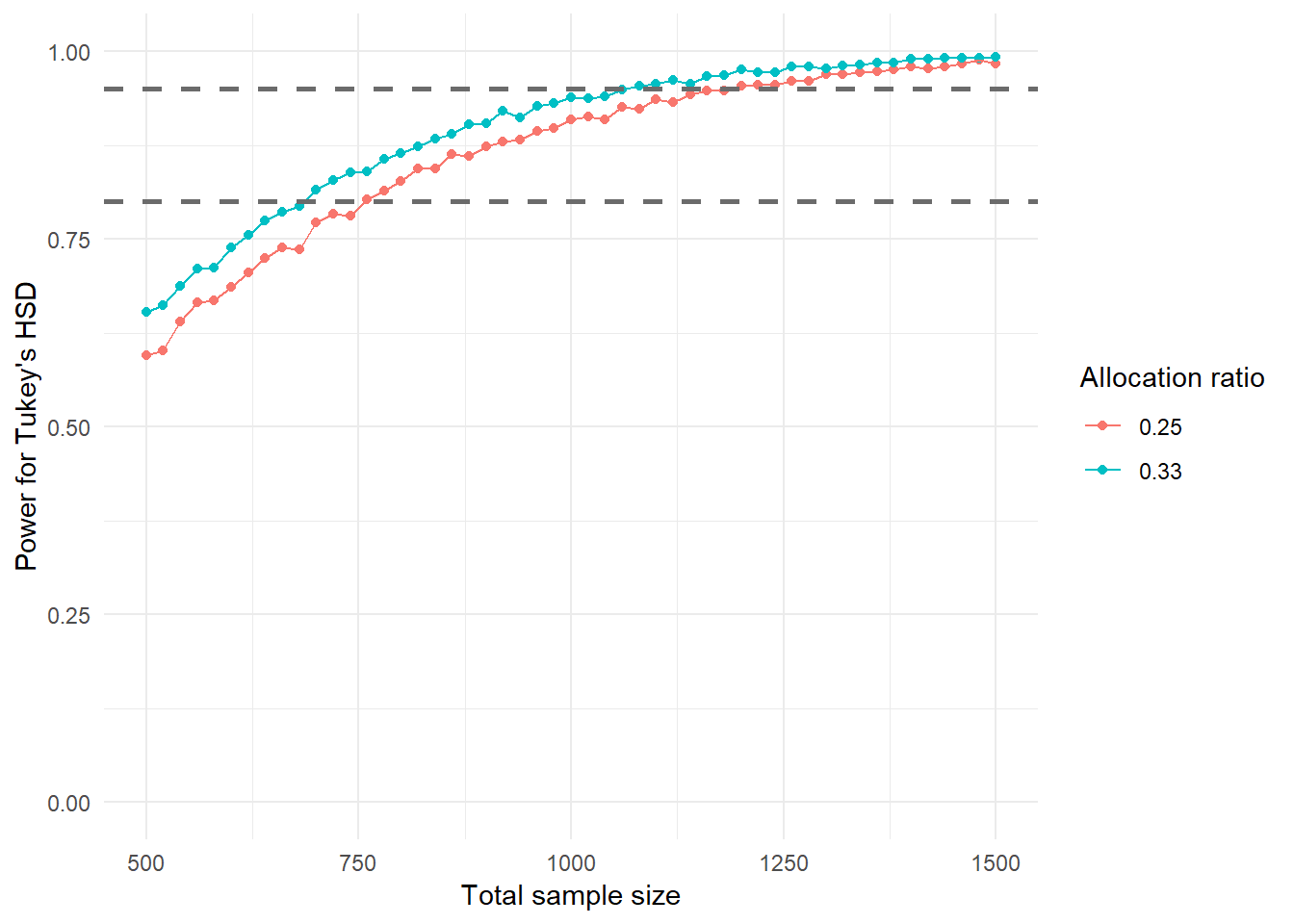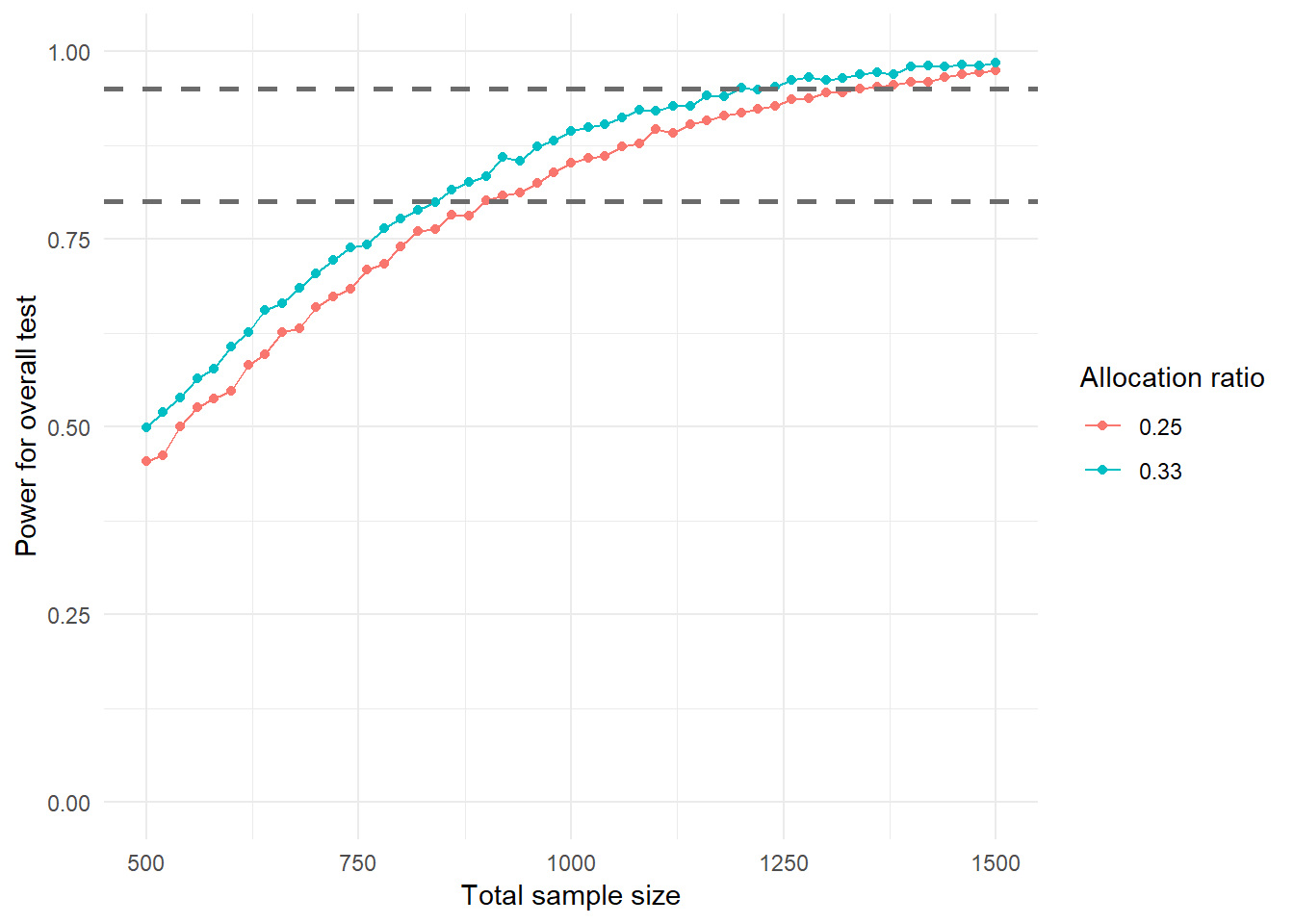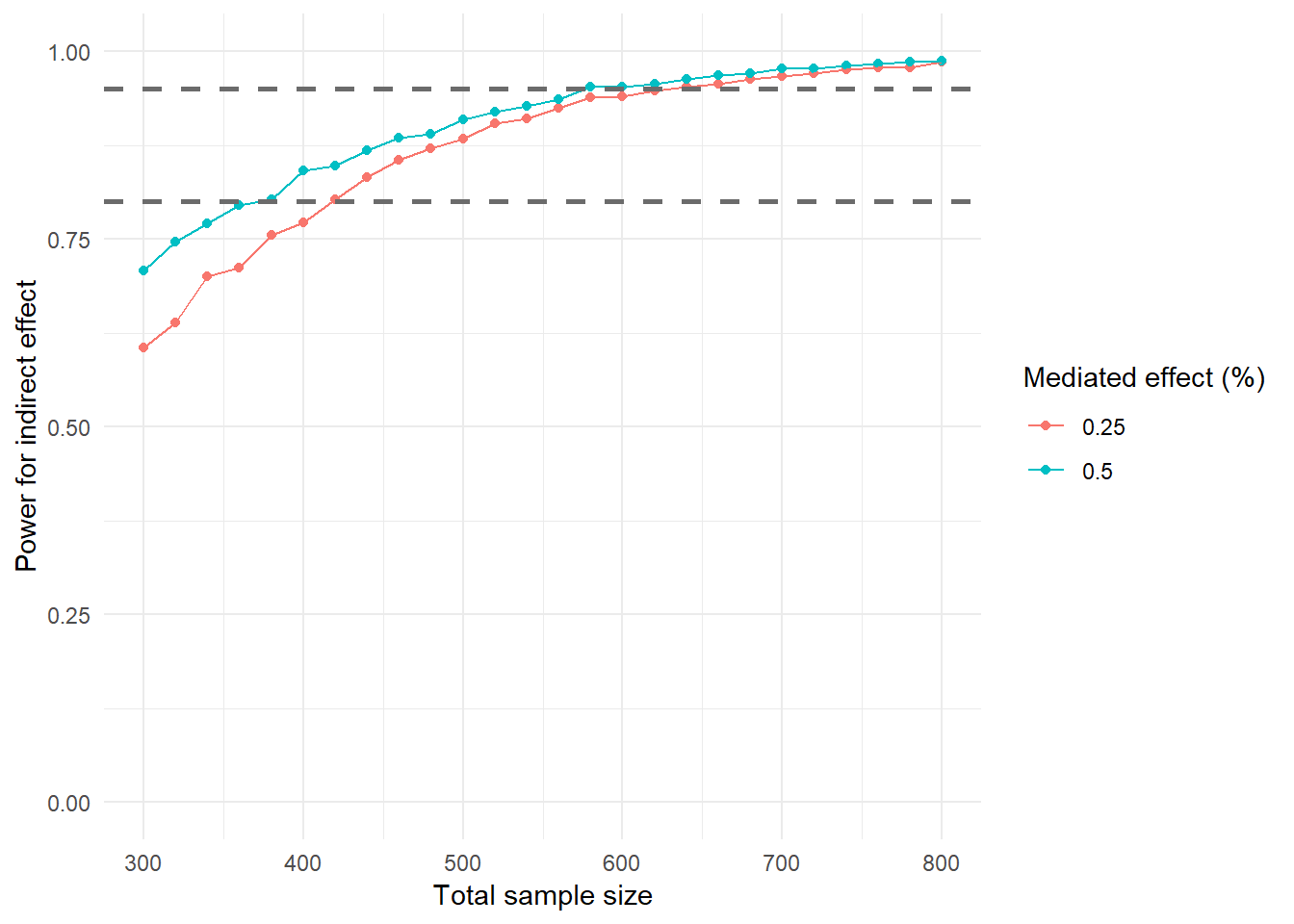Clear work space

rm(list = ls())

library(paramtest)
## Warning: package 'paramtest' was built under R version 3.5.1
library(dplyr)
## Warning: package 'dplyr' was built under R version 3.5.1
##
## Attaching package: 'dplyr'
## The following objects are masked from 'package:stats':
##
##     filter, lag
## The following objects are masked from 'package:base':
##
##     intersect, setdiff, setequal, union
library(ggplot2)
## Warning: package 'ggplot2' was built under R version 3.5.1

# (red versus gray versus green)

#
# Simulate data and analyze with F-test
# (including Tukey's HSD)
#
# @param  simNum   number of simulation
# @param  N        total sample size (for both groups)
# @param  d        effect size (Cohen's d) for men
# @param  ratio    percentage of total sample assigned to group 1
#
aov_func <- function(simNum, N, d, ratio = 0.33) {

# sample sizes for the three groups
N1 <- round(N * ratio)
N2 <- round((N - N1) / 2)
N3 <- N - N1 - N2

# draw random responses in the three groups
y1 <- rnorm(N1, d, 1)
y2 <- rnorm(N2, 0, 1)
y3 <- rnorm(N3, 0, 1)

# create data
data <- data.frame(y = c(y1, y2, y3),
grp = factor(c(rep(0, N1),
rep(1, N2),
rep(2, N3))))

# ANOVA on simulated data
mod_aov <- aov(y ~ grp, data = data)
mod_hsd <- TukeyHSD(mod_aov)$grp p_aov <- summary(mod_aov)[]["grp", "Pr(>F)"] p_hsd1 <- mod_hsd["1-0", "p adj"] p_hsd2 <- mod_hsd["2-0", "p adj"] # significance of results sig_aov <- as.numeric(p_aov < 0.05) sig_hsd1 <- as.numeric(p_hsd1 < 0.05) sig_hsd2 <- as.numeric(p_hsd2 < 0.05) sig_all <- (sig_aov == 1) & (sig_hsd1 == 1) & (sig_hsd2 == 1) & sign(mean(y1) - mean(y2)) == sign(d) & sign(mean(y1) - mean(y3)) == sign(d) # return results return(c(sig_aov = sig_aov, sig_hsd1 = sig_hsd1, sig_hsd2 = sig_hsd2, sig_all = sig_all)) } Simulate power power_aov <- grid_search(aov_func, params = list(N = seq(500, 1500, 20), ratio = c(0.25, 0.33)), n.iter = 5000, output = 'data.frame', d = 0.30, parallel = 'snow', ncpus = 4) ## Running 510,000 tests... power_aov_grp <- results(power_aov) %>% group_by(N.test, ratio.test) %>% summarise(power_aov = mean(sig_aov), power_hsd = mean(sig_hsd1), power_all = mean(sig_all)) Plot results for ANOVA main effect ggplot(power_aov_grp, aes(x = N.test, y = power_aov, group = factor(ratio.test), colour = factor(ratio.test))) + geom_point() + geom_line() + geom_hline(yintercept = .95, linetype = "dashed", color = "gray42", size = 1) + geom_hline(yintercept = .80, linetype = "dashed", color = "gray42", size = 1) + ylim(c(0, 1)) + labs(x = 'Total sample size', y = 'Power for ANOVA main effect', colour = "Allocation ratio") + theme_minimal()Plot results for Tukey’s HSD ggplot(power_aov_grp, aes(x = N.test, y = power_hsd, group = factor(ratio.test), colour = factor(ratio.test))) + geom_point() + geom_line() + geom_hline(yintercept = .95, linetype = "dashed", color = "gray42", size = 1) + geom_hline(yintercept = .80, linetype = "dashed", color = "gray42", size = 1) + ylim(c(0, 1)) + labs(x = 'Total sample size', y = "Power for Tukey's HSD", colour = "Allocation ratio") + theme_minimal()Plot results for overall test ggplot(power_aov_grp, aes(x = N.test, y = power_all, group = factor(ratio.test), colour = factor(ratio.test))) + geom_point() + geom_line() + geom_hline(yintercept = .95, linetype = "dashed", color = "gray42", size = 1) + geom_hline(yintercept = .80, linetype = "dashed", color = "gray42", size = 1) + ylim(c(0, 1)) + labs(x = 'Total sample size', y = "Power for overall test", colour = "Allocation ratio") + theme_minimal()Minimum sample sizes by conditions power_aov_grp %>% filter(power_aov >= 0.95) %>% group_by(ratio.test) %>% summarise(N = min(N.test)) ## # A tibble: 2 x 2 ## ratio.test N ## <dbl> <dbl> ## 1 0.25 920 ## 2 0.33 780 power_aov_grp %>% filter(power_hsd >= 0.95) %>% group_by(ratio.test) %>% summarise(N = min(N.test)) ## # A tibble: 2 x 2 ## ratio.test N ## <dbl> <dbl> ## 1 0.25 1200 ## 2 0.33 1080 power_aov_grp %>% filter(power_all >= 0.95) %>% group_by(ratio.test) %>% summarise(N = min(N.test)) ## # A tibble: 2 x 2 ## ratio.test N ## <dbl> <dbl> ## 1 0.25 1340 ## 2 0.33 1200 # Mediating effect of worries # # Simulate data and conduct mediation analysis # # @param simNum number of simulation # @param N total sample size (for both groups) # @param total total effect x on y # @param mediated percentage of the total effect that is mediated # @param ratio percentage of the total sample size assigned to group 1 # med_func <- function(simNum, N, total, mediated, ratio = 0.33) { # effects aa <- total # it is assumed that color yields identical # effects on cognitive test results and worries ab <- total * mediated # indirect effect bb <- ab / aa # effect of worries on test results cc <- total - ab # remaining direct effect # sample sizes for the two groups N1 <- round(N * ratio) N2 <- N - N1 # generate data x <- c(rep(0, N1), rep(1, N2)) m <- rnorm(N, aa*x, sqrt(1 - aa^2)) y <- rnorm(N, cc*x + bb*m, sqrt(1 - cc^2 - bb^2)) data <- data.frame(x, m, y) # model mod <- ' m ~ a*x y ~ c*x + b*m ab := a*b total := c + (a*b)' # SEM on simulated data fit <- lavaan::sem(mod, data = data) est <- lavaan::parameterEstimates(fit) p_med <- est[est$label == "ab", "pvalue"]

# return results
return(c(p = p_med, sig = (p_med < .05)))
}

Simulate power

power_med <- grid_search(med_func,
params = list(N = seq(300, 800, 20),
mediated = c(0.25, 0.50)),
n.iter = 5000, output = 'data.frame',
total = 0.30, ratio = 0.33,
parallel = 'snow', ncpus = 4)
## Running 260,000 tests...
power_med_grp <- results(power_med) %>%
group_by(N.test, mediated.test) %>%
summarise(power = mean(sig))

Plot results

ggplot(power_med_grp,
aes(x = N.test, y = power,
group = factor(mediated.test),
color = factor(mediated.test))) +
geom_point() +
geom_line() +
geom_hline(yintercept = .95, linetype = "dashed",
color = "gray42", size = 1) +
geom_hline(yintercept = .80, linetype = "dashed",
color = "gray42", size = 1) +
ylim(c(0, 1)) +
labs(x = 'Total sample size',
y = 'Power for indirect effect',
color = "Mediated effect (%)") +
theme_minimal()Minimum sample sizes by conditions

power_med_grp %>%
filter(power >= 0.95) %>%
group_by(mediated.test) %>%
summarise(N = min(N.test))
## # A tibble: 2 x 2
##   mediated.test     N
##           <dbl> <dbl>
## 1          0.25   640
## 2          0.5    580
power_med_grp %>%
filter(power >= 0.80) %>%
group_by(mediated.test) %>%
summarise(N = min(N.test))
## # A tibble: 2 x 2
##   mediated.test     N
##           <dbl> <dbl>
## 1          0.25   420
## 2          0.5    380

# Session info

sessionInfo()
## R version 3.5.0 (2018-04-23)
## Platform: i386-w64-mingw32/i386 (32-bit)
## Running under: Windows 10 x64 (build 17134)
##
## Matrix products: default
##
## locale:
##  LC_COLLATE=German_Germany.1252  LC_CTYPE=German_Germany.1252
##  LC_MONETARY=German_Germany.1252 LC_NUMERIC=C
##  LC_TIME=German_Germany.1252
##
## attached base packages:
##  stats     graphics  grDevices utils     datasets  methods   base
##
## other attached packages:
##  bindrcpp_0.2.2  ggplot2_3.1.0   dplyr_0.7.8     paramtest_0.1.0
##
## loaded via a namespace (and not attached):
##   fansi_0.4.0      digest_0.6.18    htmltools_0.3.6  R6_2.3.0
##   scales_1.0.0     assertthat_0.2.0 rprojroot_1.3-2  grid_3.5.0
##   utf8_1.1.4       stringr_1.3.1    knitr_1.20       cli_1.0.1
##  tidyselect_0.2.5 munsell_0.5.0    pillar_1.3.0     compiler_3.5.0
##  tibble_1.4.2     pkgconfig_2.0.2  labeling_0.3     purrr_0.2.5
##  plyr_1.8.4       glue_1.3.0       stringi_1.2.4    magrittr_1.5
##  rmarkdown_1.10   evaluate_0.12    gtable_0.2.0     rlang_0.3.0.1
##  colorspace_1.3-2 yaml_2.2.0       tools_3.5.0      parallel_3.5.0
##  bindr_0.1.1      withr_2.1.2      lazyeval_0.2.1   crayon_1.3.4
##  backports_1.1.2  Rcpp_1.0.0